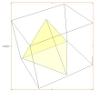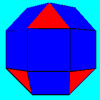#### You may also like### A Mean Tetrahedron

Can you number the vertices, edges and faces of a tetrahedron so that the number on each edge is the mean of the numbers on the adjacent vertices and the mean of the numbers on the adjacent faces?### Rhombicubocts

Each of these solids is made up with 3 squares and a triangle around each vertex. Each has a total of 18 square faces and 8 faces that are equilateral triangles. How many faces, edges and vertices does each solid have?### Icosian Game

This problem is about investigating whether it is possible to start at one vertex of a platonic solid and visit every other vertex once only returning to the vertex you started at.

# Triangles to Tetrahedra

##### Age 11 to 14Challenge Level

Think about how the lengths of sides of the triangles are important.

You could use Polydron (plastic shapes that click together) to make the models yourself.